[洛尔定理]  如果(i)函数f(x)定义在闭区间[a,b]上而且是连续的,(ii)在开区间(a,b)内存在有限导数,(iii)在区间的两端点处函数值相等: f (a)= f (b).那末在ab之间至少存在一点c,使=0.即曲线y= f (x)在点(c, f (c))处的切线是水平的(5.6).

f (x)=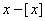在区间[0,1],除去在x=1时有间断以外满足定理的一切条件,但在(0,1)内处处都是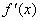=1.又例如由等式f (x)=x(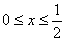)f (x)=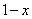()所定义的函数,在这区间内除去当x=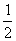(双边的)导数不存在以外,它也满足定理的一切条件,可是导数在左半区间内等于+1,而在右半区间内等于.

[中值定理]  如果(i) f (x)定义在闭区间[a,b]上而且是连续的,(ii) 在开区间(a,b)内存在有限导数,那末在ab之间至少存在一点c,满足等式

`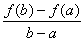=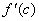(a<c<b)                                            (1)`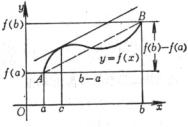图5.7

(1)式也常写成以下几种形式:

f (b)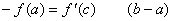f (x+Δx)Δx    (x<c<x+Δx)

Δy= f (x+Δx)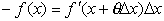(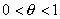)

[柯西定理]  如果(i)函数f(t)g(t)在闭区间[a,b]上连续,(ii)在开区间(a,b)内有有限导数,(iii)在区间(a,b)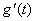0.那末在ab之间至少存在一点c,使图5.8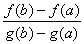=(a<c<b)

[多变量函数的中值定理]  如果(i)函数f(x,y)定义在闭区域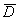上并且连续,(ii)在这区域内部(即在它的所有内点)有连续的偏导数,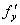,今考察D中的两点

M0(x0,y0)M1(x0+Δx,y0+Δy)

Δf(x0,y0)=f(x0+Δx,y0+Δy)=(0<θ<1)==0,*  若区域的任意两点可以用一“折线”来连接，而该折线的一切点都在这区域中，这区域就称为连通区域.